# How to set encoder offset

Motor control often relies on using the rotating dq-reference frame. The rotating dq-reference frame for permanent magnet synchronous machines (PMSM) is aligned with the d-axis and relies on the knowledge of the angle $$\vartheta_{el}$$ between d-axis and $$\alpha$$ axis. The angle $$\vartheta_{el}$$ can be measured or estimated and is used in the transformation, e.g., using Coordinate Transformation. Additionally, machine models in dq-frame assume this alignment. Several different encoder technologies exist, e.g., Incremental Encoder. The encoder reference (the zero-point of the encoder) is not necessarily aligned with the d-axis of the machine.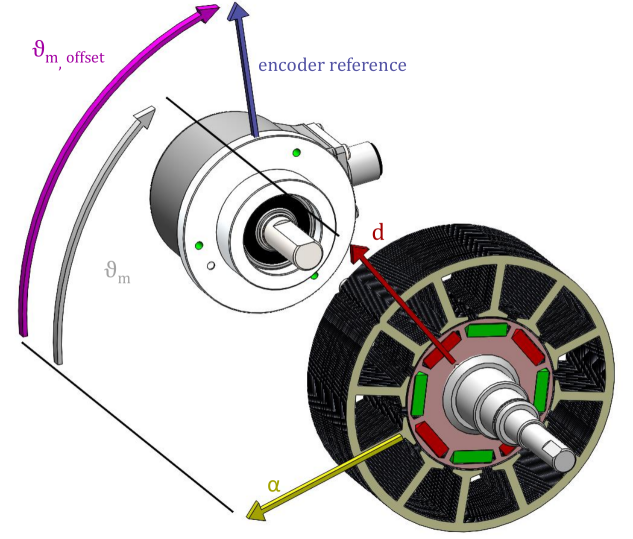Fig. 37 Mechanical alignment of the encoder with respect to the $$\alpha$$-axis and d-axis

This alignment is shown in fig. Fig. 37 with the angle $$\vartheta_m$$ between the $$\alpha$$-axis and the d-axis and the mechanical encoder offset $$\vartheta_{m,offset}$$ between the $$\alpha$$-axis of the machine and the encoder reference. Note that a machine with $$p$$ pole pairs has $$p$$ different d-axis (each with a positive and negative pole). While the encoder measures the mechanical angle $$\vartheta_m$$ of the machine , the electrical angle $$\vartheta_{el}$$ is required for the dq-transformations. The electrical angle is calculated using the modulo operation:

$\vartheta_{el} = (\vartheta_m \cdot p - \vartheta_{el,offset}) \bmod 2\pi$

With the encoder offset in the electrical radian $$\vartheta_{el,offset}$$ assuming $$\vartheta_m$$ is measured in radian and one mechanical rotation is equal to $$\vartheta_m=2\pi$$. The usage of the offset $$\vartheta_{el,offset}$$ is assumed in the subsequent sections. The following methods for determining the offset are commonly used for PMSM:

## Constant current in the a-phase

The linked flux of the $$a$$-phase winding is aligned with the $$\alpha$$-axis of the machine. The main idea in this offset estimation is to drive a constant current through the $$a$$-phase of the machine, which generates a linked magnetic flux leading the d-axis with the permanent magnet to mechanically turn and align with the $$\alpha$$-axis of the machine. This can be achieved by stepping the duty cycle of the $$a$$-phase from zero to an arbitrary duty cycle. The duty cycle is kept on its value and the current angle $$\vartheta_{el}$$ is set as $$\vartheta_{el,offset}$$. Since the method does not use a closed-loop current control, a duty cycle has to be chosen that does not lead to a-phase current above the rated current of the machine. However, the duty cycle has to be sufficiently large to move the rotor reliably into the $$\alpha$$ axis. This method is quick but results in sub-optimal alignment, requires manual tuning of the duty cycle, and is often unreliable in the laboratory. Additionally, $$L_d=L_q$$ is assumed.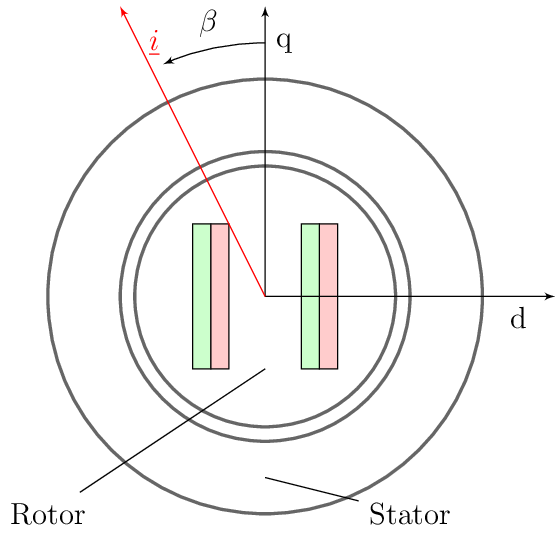Fig. 38 dq-reference frame in PMSM based on [3, p. 7]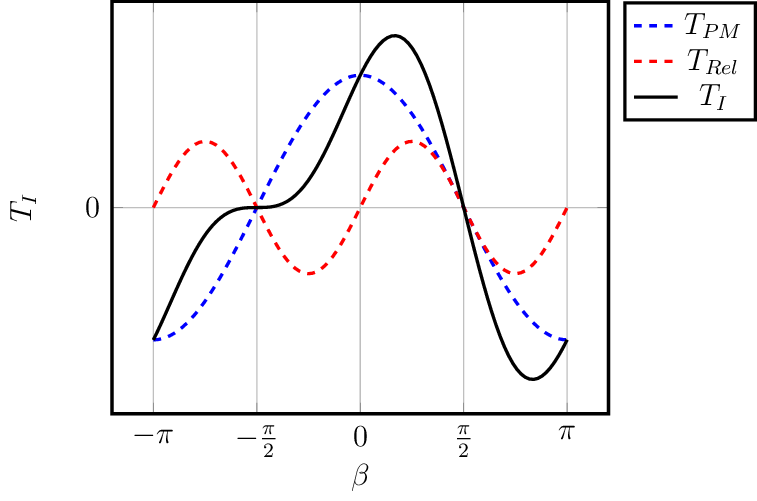Fig. 39 Torque components over current angle based on [3, p. 7]

The figure left showcases the relationship between the current angle $$\beta$$ and machine torque with the torque generated by the reluctance effect $$T_{Rel}$$, the torque generated by the linked flux of the permanent magnet $$T_{PM}$$ and the sum of both torque components $$T_{I}$$ (the inner torque of the machine). The current angle $$\beta$$ describes the angle between the q-axis and the space vector of the stator current $$\underline{i}=i_d + ji_q$$ (with the imaginary unit $$j$$). Therefore, $$\beta=\arctan{\big( \frac{-i_d}{i_q} \big) }$$. For machines with $$L_d=L_q$$ only the blue torque curve applies. Therefore, current in the $$a$$-phase prompts the rotor to move into the $$\alpha$$-axis aligning the peak of the torque with the d-axis. If $$L_d \neq L_q$$, the blue curve is superimposed with the red curve yielding the resulting torque (black line). Thus, current in the $$a$$-phase does not align the $$\alpha$$-axis with the d-axis but with an angle that is skewed depending on the specific values of the inductances $$L_d$$, $$L_q$$, and flux linkage of the permanent magnet $$\psi_{PM}$$. The method for determining the encoder offset $$\vartheta_{el,offset}$$ must not be used on a machine with $$L_d \neq L_q$$.

• Quick & easy

• Assumes $$L_d = L_q$$

• Manual estimation of end-value for duty cycle step (high enough to move the rotor, not too high to damage machine)

• No closed-loop control to prevent exceeding the rated current of the machine

## No-torque by d-current

A possible alternative for driving current through the $$a$$-phase of the machine is to ensure that no torque is generated if $$i_q=0$$ and $$i_d \neq 0$$ since the PMSM does not generate torque in this case [1,p. 1092]:

$T_I=\frac{3}{2} p \big(i_q \psi_{pm} + i_d i_q (L_d -L_q) \big)$

This alignment method uses a closed-loop current control and control the set-points $$i_q^*=0$$ and $$i_d^* \neq 0$$. Using a torque sensor on the test bench (as described in ), the encoder offset can manually be adjusted until the torque sensor measures zero torque. The set-point for the current $$i_d$$ depends on the machine under test.

• Simple concept

• Manual tuning

• Requires torque sensor

• Suitable for machines with $$L_d = L_q$$ and $$L_d \neq L_q$$

• Not suitable for synchronous reluctance machines with $$\psi_{PM}=0\,Vs$$ (since neither $$I_d$$ nor $$I_q$$ generates torque if the other current is zero)

• The accuracy of the torque sensor determines the accuracy of the encoder alignment

## Induced voltage (back-EMF)

Alignment of the encoder and the d-axis can be achieved if the zero-crossing of the induced voltage $$u_i=\omega_{el}\psi_{PM}$$ (back-EMF) and the position signal is aligned as shown in Fig. 40 . However, this method for determining the encoder offset $$\vartheta_{el,offset}$$ requires that the machine under test is driven by an external machine to a fixed rotational speed and the induced voltage, as well as the encoder signal, are measured by one oscilloscope or the controller. The machine under test is operated with open circuit, i.e., $$i_d=i_q=0$$. If the induced voltage should be measured by the controller, a phase voltage measurement is required on the power electronics. Voltage measurement on the power electronics is not always available and if it is, usually a low pass filter is included which adds a phase lag to the measured voltage which has to be accounted for when aligning the encoder. Furthermore, the position alignment does not account for iron losses.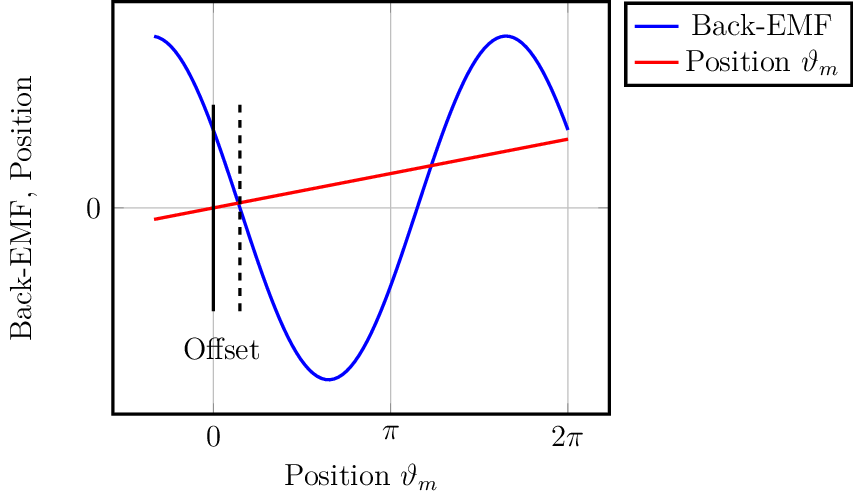Fig. 40 Alignment of zero-crossing of induced voltage with zero-crossing of encoder position 

• Complex setup with encoder signal on external measurement device (e.g., oscilloscope) or voltage measurement on inverter

• Does not account for iron losses. This leads to misalignment since the linked flux is not solely based on the induced voltage of the permanent magnet but also generated by the iron loss current (see Flux-based (without test-bench machine)).

## Flux-based (with test-bench machine)

The flux-based encoder alignment is based on the induced voltage (back-EMF) and uses the same operating condition as the back-EMF-based method. The machine under test for which the encoder offset should be determined is driven by a prime mover (e.g., a test bench machine)to a constant speed (open circuit, thus $$I_d=I_q=0$$). In steady state, the voltage equations of the PMSM are given by [4,p. 16]:

\begin{split}\begin{align} U_d &=R_1 I_d - \omega_{el} L_q I_q \\ U_q &=R_1 I_q + \omega_{el} L_d I_d + \omega_{el} \psi_{PM} \end{align}\end{split}

With $$I_d=0$$ and $$I_q=0$$ due to open circuit, the equations simplify to:

\begin{split}\begin{align} U_d &=0 \\ U_q &=0 + \omega_{el} \psi_{PM} \end{align}\end{split}

Based on these equations, encoder alignment is reached for an offset $$\vartheta_{el,offset}$$ in which $$U_d=0$$ and $$U_q=\omega_{el} \psi_{PM}$$ is measured. However, this approach does not work due to iron losses in the machine and the alignment is skewed if this method is used.Fig. 41 Equivalent circuit of PMSM including iron losses of d-axis (left) and q-axis (right) [4, p. 71, 1, p. 1102].

Fig. 41 shows the equivalent circuit of the PMSM including iron losses. With the parallel iron resistance $$R_c$$, the equations of the PMSM in steady state can be written as:

$\begin{split}U_d &=R_1 I_d + R_c I_{dc} \\ U_q &=R_1 I_q + R_c I_{qc} \\ I_d &=I_{dc}+I_{d0} \\ I_q &=I_{qc}+I_{q0}\end{split}$

Simplifying the equations by using open circuit operation with $$I_d=I_q=0$$ and $$\omega_{el}\neq 0$$:

$\begin{split}U_d &=R_c I_{dc} =-\omega_{el} L_q I_{q0}\\ U_q &=R_c I_{qc} = \omega_{el} \psi_{PM} + \omega_{el} L_d I_{d0}\\ I_{dc} &= -I_{d0} \\ I_{qc} &= -I_{q0}\end{split}$

Following the equivalent circuit and the equations, the induced voltage $$\omega_{el}\psi_d$$ leads to the iron loss current $$I_{q0}$$ and the current $$I_{q0}$$ generates the flux linkage $$-\omega_{el} \psi_q$$ [5, p. 44]. If the dq-frame is aligned with the d-axis, the induced voltage in the d-axis $$U_d \neq 0$$ according to Fig. 41. Therefore, the encoder offset to match $$U_d=0$$ and $$U_q=\omega_{el} \psi_{PM}$$ does not lead to an alignment of the dq-frame to the d-axis in the aforementioned operating conditions. Instead, the encoder offset $$\vartheta_{el}$$ has to be determined for positive and negative rotational speeds accounting for the effect of the iron losses as discussed. The dq-frame is aligned with the d-axis if $$U_q$$ changes the sign for positive and negative rotational speed but not its magnitude and the value for $$U_d$$ does not change when changing the direction.

To simplify the method, a closed-loop current control with set points $$I_q^*=0$$ and $$I_d^*=0$$ can be used instead of open circuit. The following steps have to be performed to align the encoder and determine $$\vartheta_{el,offset}$$:

1. Machine coupled with test bench machine

2. Closed-loop current control with set points $$I_q^*=0$$ and $$I_d^*=0$$

3. Measure $$U_d$$ and $$U_q$$ or measure controller outputs $$U_d^*$$ and $$U_q^*$$

4. Set test bench machine to some $$\omega \neq 0$$ within the operating range and alternate between positive and negative rotation

5. Adjust encoder offset $$\vartheta_{el,offset}$$ until $$U_{d,\omega > 0}=U_{d,\omega < 0}$$ and $$U_{q,\omega > 0}= - U_{q,\omega < 0}$$

This method yields good results for determining the encoder offset but requires a test-bench machine.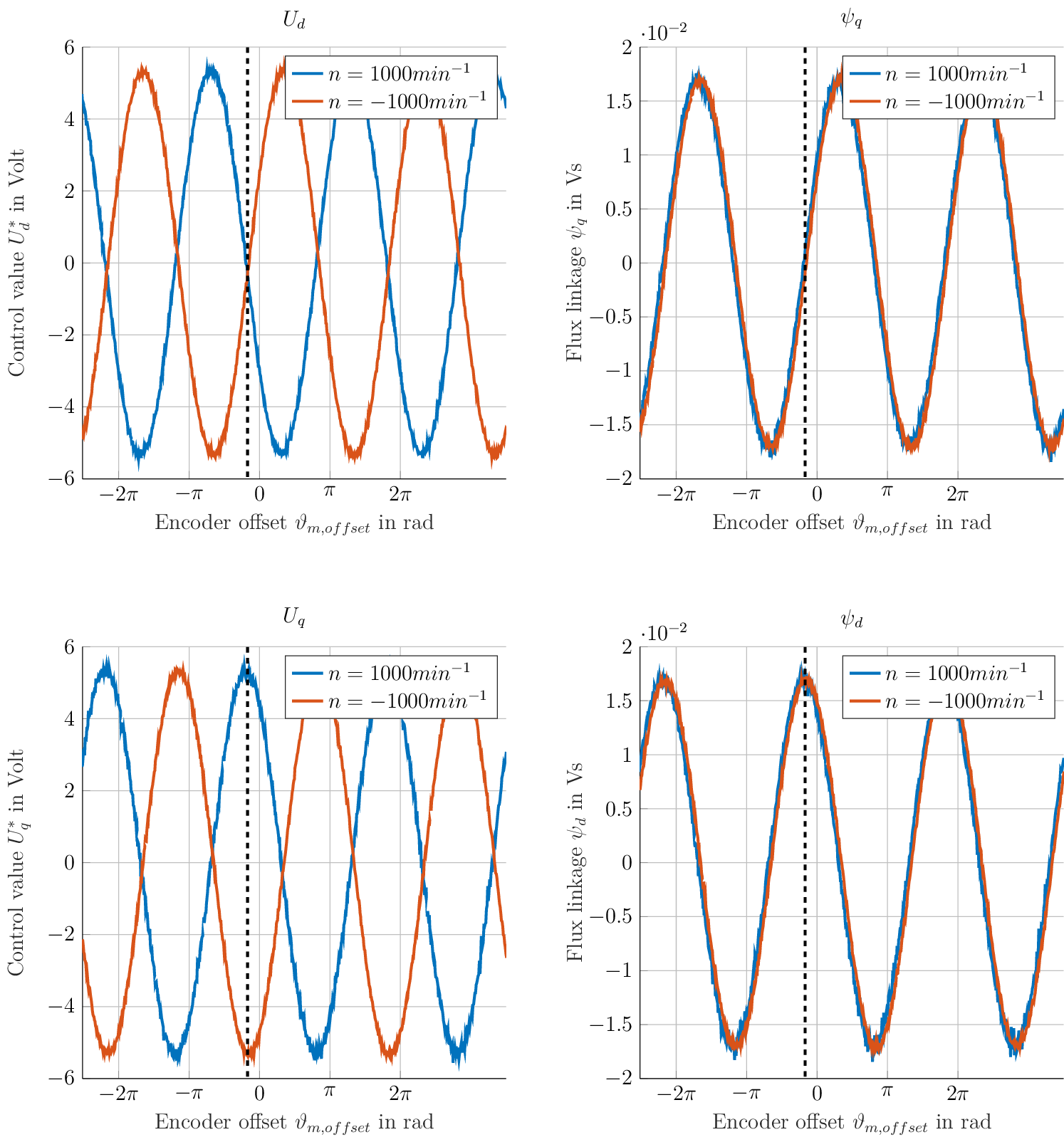Fig. 42 Measurement results of Heidrive HDM06-005 based on the control value of the d- and q-axis PI-controller with constant speed $$n=1000 min^{-1}$$

Fig. 42 shows measurement results for the voltages (control value of the current controller) $$U_d^*$$, $$U_q^*$$ and the calculated flux linkage $$\psi_d=\frac{U_q^*}{\omega_{el} }$$, $$\psi_q=\frac{U_d^*}{-\omega_{el} }$$.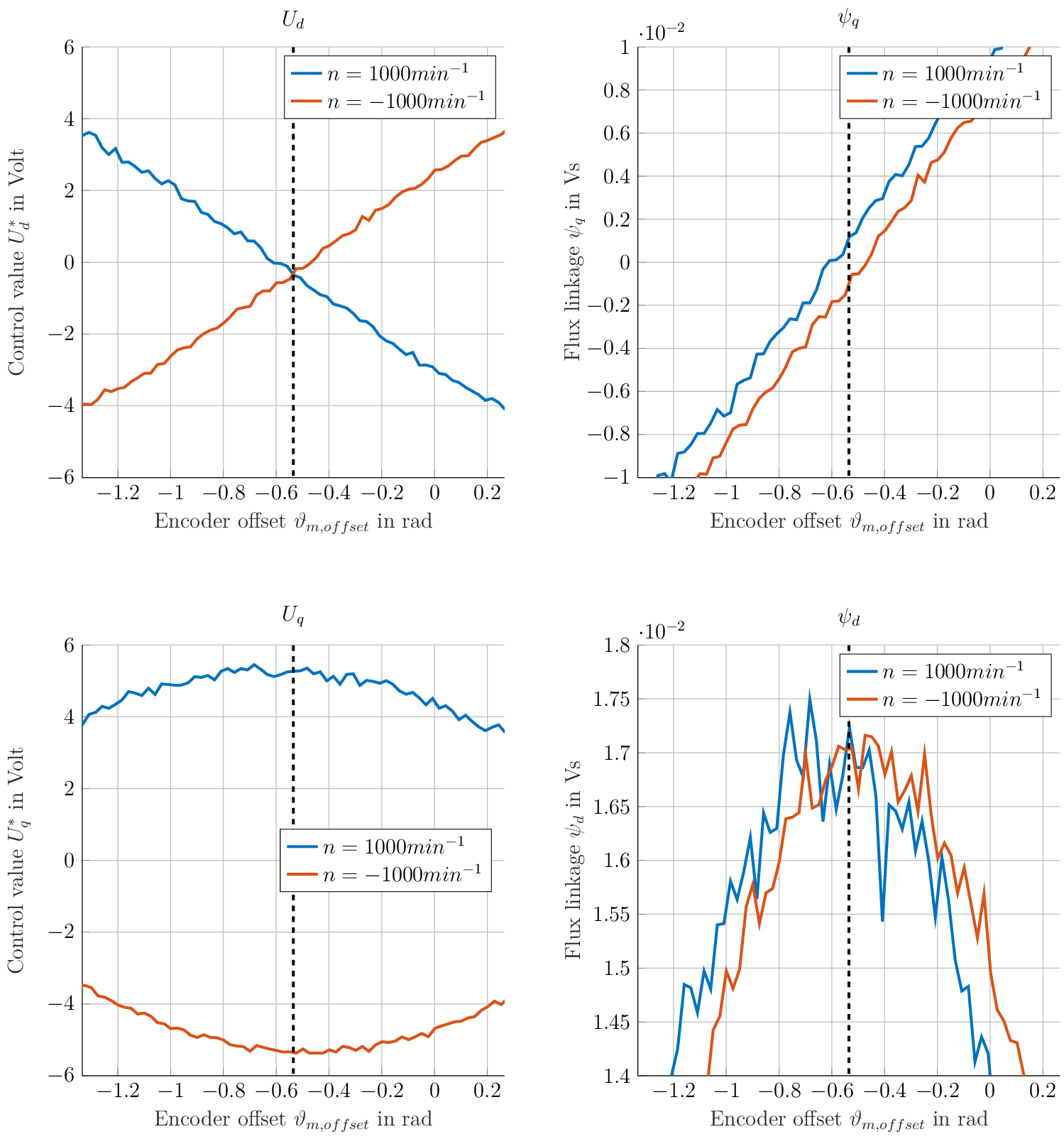Fig. 43 Measurement results of Heidrive HDM06-005 based on the control value of the d- and q-axis PI-controller with constant speed $$n=1000 min^{-1}$$

Fig. 43 shows a section of the measurement to highlight the described point in which the dq-frame is aligned (dashed line).

## Flux-based (without test-bench machine)

The concept of the flux-based alignment can be extended to not require a test-bench with a load machine. The basic principle stays the same regarding the aim of the encoder offset, i.e., $$U_{d,\omega > 0}=U_{d,\omega < 0}$$ and $$U_{q,\omega > 0}= - U_{q,\omega < 0}$$. However, instead of using a load machine to keep the machine for which the encoder offset should be determined at a constant rotational speed the machine itself is controlled to a rotational speed, then the currents in d- and q-axis are controlled to zero $$I_q^*=0$$ and $$I_d^*=0$$ and the measurement is automatically done during run out of the machine.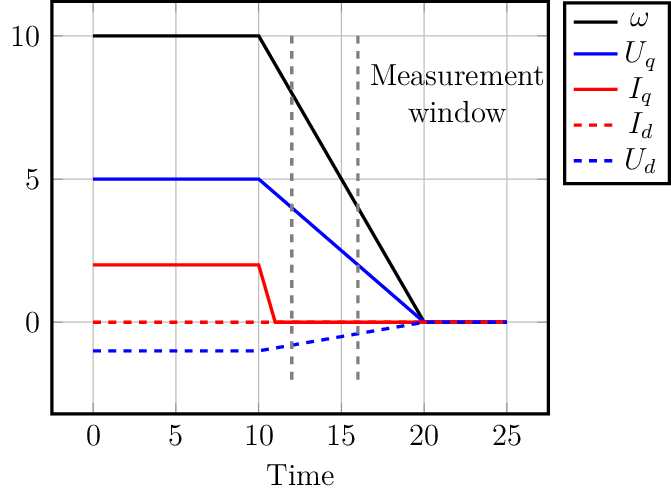Fig. 44 Principle of the measurement during run out of the machine

Fig. 44 shows the basic principle of the measurement during run-out of the machine. As described in the preceding section, the control value of the current controller are used for the calculation.

$\begin{split}U_d^* &= -\omega_{el} \psi_q \\ U_q^* &= \omega_{el} \psi_d \\\end{split}$

Rearranged:

$\begin{split}\frac{U_d^*}{-\omega_{el}} &= \psi_q \\ \frac{U_q^*}{\omega_{el}} &= \psi_d\end{split}$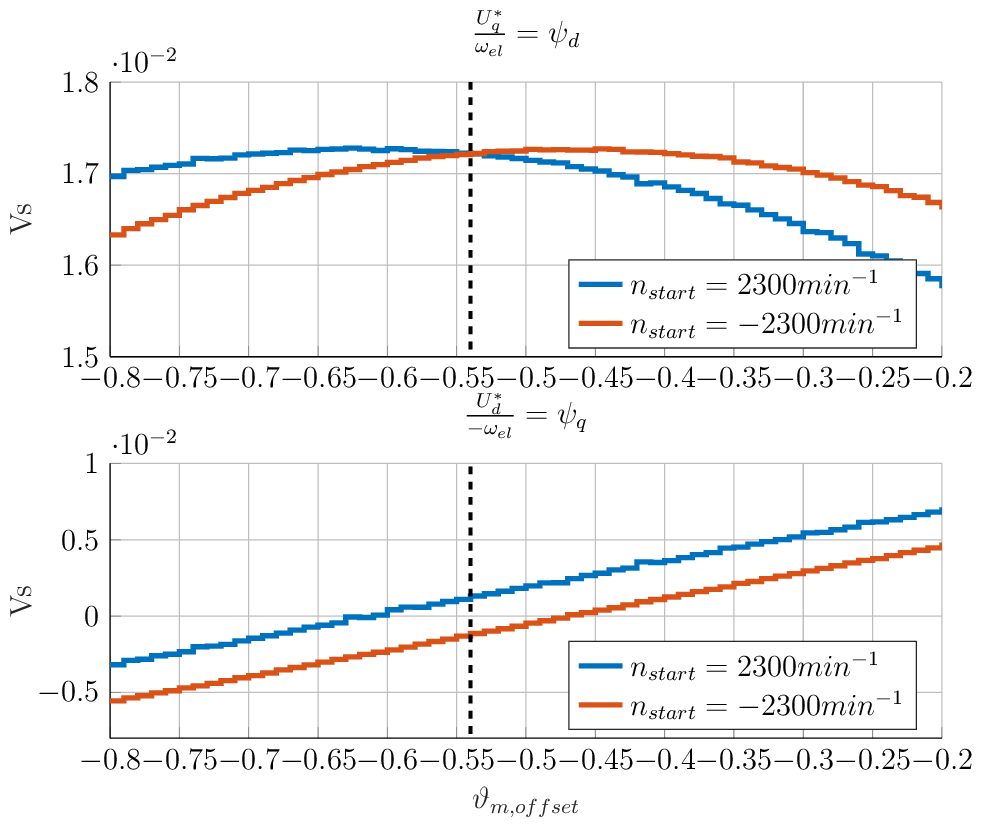Fig. 45 Measurement results of Heidrive HDM06-005 based on the control value of the d- and q-axis PI-controller with run out starting at different rotational speeds

Fig. 45 shows the measurement result for measuring in the run out of the machine with the run out starting at $$n=2300\,min^{-1}$$ and $$n=-2300\,min^{-1}$$.

## Sources

1(1,2)

Elektrische Antriebe - Regelung von Antriebssystemen, Dierk Schröder, Springer, 2015, 4. Edition (German)

2(1,2,3)
1. Rahman and S. Hiti, “Identification of machine parameters of a synchronous motor,” in IEEE Transactions on Industry Applications, vol. 41, no. 2, pp. 557-565, March-April 2005, doi: 10.1109/TIA.2005.844379.

3(1,2)

Winzer, Patrick, 2017, Dissertation, “Steigerung von Drehmoment und Wirkungsgrad bei Synchronmaschinen durch Nutzung der magnetischen Asymmetrie”, DOI: 10.5445/IR/1000071097, https://publikationen.bibliothek.kit.edu/1000071097

4(1,2)

Sven Kellner, Dissertation, “Parameteridentifikation bei permanenterregten Synchronmaschinen”, Verlag Dr. Hut, ISBN 978-3-8439-0845-0, https://www.google.com/url?sa=t&rct=j&q=&esrc=s&source=web&cd=&ved=2ahUKEwja6rLEx_v6AhUEX_EDHSrRAb4QFnoECBAQAQ&url=https%3A%2F%2Fopus4.kobv.de%2Fopus4-fau%2Ffiles%2F2738%2FSvenKellnerDissertation.pdf&usg=AOvVaw3h5c9Z0-2m8zLh30i5mtz1

5

Jan Richter, Dissertation, “Modellbildung, Parameteridentifikation und Regelung hoch ausgenutzter Synchronmaschinen”, https://www.ksp.kit.edu/site/books/m/10.5445/KSP/1000057097/